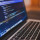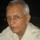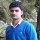Tough Puzzles & Brain Teasers
Puzzles on logic and mathematics that will make you scratch your head.
80 Members
Join this group to post and comment.Aswini Vi
Computer Science
13 Jan 2019

# If 1 1 1 1=R, 2 2 2 2=T,3 3 3 3=E, then what is 4 4 4 4=?

If 1 1 1 1=R,

2 2 2 2=T,

3 3 3 3=E, then

What 4 4 4 4 equal to?4mos ago

N

The last letter of the sum of the digits

Sum of 1,1,1,1 = fouR

Sum of 2,2,2,2=eighT

Sum of 3,3,3,3=twelvE. So,

Sum of 4,4,4,4 =sixteeNVijay Khatri
Computer Science
3mos ago

N

The last letter of the sum of the digits

Sum of

1,1,1,1 = fouR

Sum of 2,2,2,2=eighT

Sum of 3,3,3,3=twelvE.

So,

Sum of 4,4,4,4 =sixteeN

@Ramani Aswath, Has given the right answer of your questions?